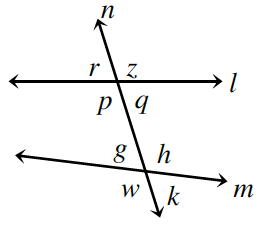### Home > CCG > Chapter 6 > Lesson 6.2.2 > Problem6-62

6-62.

Examine the diagram at right. Based on the information below, what statement can you make about the relationships between the lines? Be sure to justify each conclusion. Remember: each part below is a separate problem.1. $p=h$

When do alternate interior angles have equal measures?

If $p=h$, then $l$ and $m$ are parallel.

1. $w=k$

If two angles which form a straight angle are congruent, what must their measures be?

$n$ is perpendicular to $m$ because $w$ and $k$ are both $90º$.

1. $r=q$

Under what circumstances are vertical angles congruent?

1. $z+k=160º$

Is there anything special about angles that add up to $160º$?